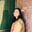Related Tags

javascript

# What are number properties in JavaScript?Umme Ammara

JavaScript has a Number object that is a wrapper for primitive numeric values. It is used to represent and manipulate numbers.

## Number properties

The standard properties of the Number object in JavaScript are as follows:

### MAX_VALUE

The MAX_VALUE property represents the largest numeric value that can be represented in JavaScript. The values that are larger than MAX_VALUE are represented as Infinity.

The code below shows the syntax and value of MAX_VALUE.

var largest_num = Number.MAX_VALUE;
console.log (largest_num);

### MIN_VALUE

The MIN_VALUE property represents the smallest numeric value that can be represented in JavaScript. The values that are smaller than MIN_VALUE are represented as negative infinity.

The code below shows the syntax and value of MIN_VALUE.

var smallest_num = Number.MIN_VALUE;
console.log (smallest_num);

### POSITIVE_INFINITY

POSITIVE_INFINITY represents the value of infinity, i.e., any value greater than MAX_VALUE. The POSITIVE_INFINITY holds the same properties as infinity in mathematics.

• Any value multiplied by POSITIVE_INFINITY returns POSITIVE_INFINITY.

• Any value divided by POSITIVE_INFINITY returns zero.

The syntax and value of POSITIVE_INFINITY are shown in the code below:

var pos_infinity = Number.POSITIVE_INFINITY;
var num = 20;
console.log (pos_infinity);
console.log(num*pos_infinity);
console.log(num/pos_infinity);

### NEGATIVE_INFINITY

NEGATIVE_INFINITY represents the value of negative infinity, i.e., any value less than MIN_VALUE.

The syntax and value of NEGATIVE_INFINITY are shown in the code below:

var neg_infinity = Number.NEGATIVE_INFINITY;
console.log (neg_infinity);


### NaN

The NaN property represents a Not-a-Number value. It is usually used for error conditions to indicate that the number is not valid.

The following code shows an example of the use of the NaN property.

var month = -1;

if (month < 1 || month > 12){
month = Number.NaN;
console.log(month);
}

Other number properties of the Number object are shown below:

 Property Description MIN_SAFE_INTEGER Represents minimum safe integer in JavaScript. MAX_SAFE_INTEGER Represents maximum safe integer in JavaScript.

RELATED TAGS

javascript

CONTRIBUTORUmme Ammara